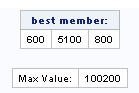## Mathematical Programming

Hello,

I was trying to solve a linear programming problem with SAS and R but got different results.

Linear Programming Problem with SAS

proc optmodel;
var x {1..3} >=0;
max z =11*x+16*x+15*x;
con c1: x+2*x+1.5*x <=12000;
con c2: 0.66*x+0.66*x+x <= 4600;
con c3: 0.5*x+0.3333*x+0.5*x <=2400;
solve with lp;print x x x;
quit;

SAS output: Objective Value=100311.708 x1=490.31 x2=5044.7 x3=946.93

With R

> library(lpSolve)

> obj=c(11,16,15)
> con=matrix(c(1,2,3/2,2/3,2/3,1,1/2,1/3,1/2),nrow=3,byrow=TRUE)
> dir=c("<=","<=","<=")
> rhs=c(12000,4600,2400)
> lp("max",obj,con,dir,rhs)
Success: the objective function is 100200
> lp("max",obj,con,dir,rhs)\$solution
 600 5100 800

1 ACCEPTED SOLUTION

Accepted SolutionsKsharp
Super User

## Re: Mathematical Programming

R is right. Maybe you should use 1/3 to replace 0.333 , maybe that is reason.

I runned it again by GA . I am astonishing that I can't use CALL LPSOLVE() . it said out of memory.

That is really weird.

``````proc iml;
start func(x) global(coef,b);
if any(coef*x`>b) then v=0;
else v=11#x+16#x+15#x;
return (v);
finish;

coef = {3   6   4.5,
2   2   3,
1.5 1   1.5}/3;
b = { 12000,4600,2400 };

id=gasetup(1,3,123456789);
call gasetobj(id,1,"func");
call gasetsel(id,100,1,0.95);
call gasetcro(id,0.05,4);
call gasetmut(id,0.05);

call gainit(id,10000,{0 0 0,10000 10000 10000});
niter = 10000;
do i = 1 to niter;
call garegen(id);
call gagetval(value, id);
end;
call gagetmem(mem, value, id, 1);
print mem[ l="best member:"],
"Max Value: " value[l = ""] ;
call gaend(id);
quit;``````9 REPLIES 9Ksharp
Super User

## Re: Mathematical Programming

R is right. Maybe you should use 1/3 to replace 0.333 , maybe that is reason.

I runned it again by GA . I am astonishing that I can't use CALL LPSOLVE() . it said out of memory.

That is really weird.

``````proc iml;
start func(x) global(coef,b);
if any(coef*x`>b) then v=0;
else v=11#x+16#x+15#x;
return (v);
finish;

coef = {3   6   4.5,
2   2   3,
1.5 1   1.5}/3;
b = { 12000,4600,2400 };

id=gasetup(1,3,123456789);
call gasetobj(id,1,"func");
call gasetsel(id,100,1,0.95);
call gasetcro(id,0.05,4);
call gasetmut(id,0.05);

call gainit(id,10000,{0 0 0,10000 10000 10000});
niter = 10000;
do i = 1 to niter;
call garegen(id);
call gagetval(value, id);
end;
call gagetmem(mem, value, id, 1);
print mem[ l="best member:"],
"Max Value: " value[l = ""] ;
call gaend(id);
quit;``````## Re: Mathematical Programming

I got the same answer by chaning 0.33 to 1/3.

Thank you.

## Re: Mathematical Programming

And also change 0.66 to 2/3.

## Re: Mathematical Programming

Can you please post the IML code for which LPSOLVE yielded out of memory?Ksharp
Super User

## Re: Mathematical Programming

```Rob,
Here is :

proc iml;

object = { 11 16 15 };
coef = {3   6   4.5,
2   2   3,
1.5 1   1.5}/3;
b = { 12000,4600,2400 };

rowsense = {'L','L','L'};
cntl= {-1,1};
call lpsolve (rc,objv,x,dual,rd,object,coef,b,cntl,rowsense);
print objv, x, dual, rd,rc;

quit;

NOTICE: in output , RC=5 , which means lpsolve() can't get enough memory.

1          OPTIONS NONOTES NOSTIMER NOSOURCE NOSYNTAXCHECK;
51
52         proc iml;
53
54         object = { 11 16 15 };
55         coef = {3   6   4.5,
56                 2   2   3,
57                 1.5 1   1.5}/3;
58         b = { 12000,4600,2400 };
59
60         rowsense = {'L','L','L'};
61         cntl= {-1,1};
62         call lpsolve (rc,objv,x,dual,rd,object,coef,b,cntl,rowsense);
ERROR: Out of memory.
NOTE: Number of iterations = 0.
NOTE: Time used (secs) = 0.00.
63         print objv, x, dual, rd,rc;
64
65         quit;
NOTE: Exiting IML.
NOTE: PROCEDURE IML used (Total process time):
real time           0.34 seconds
cpu time            0.08 seconds

```

## Re: Mathematical Programming

Hmm, that is strange.  Here's what I get with SAS/IML 14.1 on my Windows laptop:

1 option fullstimer;
2
3 proc iml;
NOTE: Writing HTML Body file: sashtml.htm
4
5 object = { 11 16 15 };
6 coef = {3 6 4.5,
7 2 2 3,
8 1.5 1 1.5}/3;
9 b = { 12000,4600,2400 };
10
11 rowsense = {'L','L','L'};
12 cntl= {-1,1};
13 call lpsolve (rc,objv,x,dual,rd,object,coef,b,cntl,rowsense);
NOTE: The LP solver is called.
NOTE: The Dual Simplex algorithm is used.
NOTE: Optimal.
NOTE: Objective value = -100200.
NOTE: Number of iterations = 5.
NOTE: Time used (secs) = 0.00.
14 print objv, x, dual, rd,rc;
15
16 quit;
NOTE: Exiting IML.
NOTE: PROCEDURE IML used (Total process time):
real time 0.73 seconds
user cpu time 0.39 seconds
system cpu time 0.21 seconds
memory 14412.64k
OS Memory 23248.00k
Timestamp 07/02/2016 09:37:55 AM
Step Count 1 Switch Count 0

What release are you running?Ksharp
Super User

## Re: Mathematical Programming

I am using SAS University Edition.

## Re: Mathematical Programming

attachments. -##

I am using SAS on demand for academic s.Ksharp
Super User

## Re: Mathematical Programming

```Here is right code :

proc optmodel;
var x {1..3} >=0;
max z =11*x+16*x+15*x;
con c1: x+2*x+1.5*x <=12000;
con c2: 2/3*x+2/3*x+x <= 4600;
con c3: 0.5*x+1/3*x+0.5*x <=2400;
solve with lp;print x x x;
quit;

```
Discussion stats
• 9 replies
• 1305 views
• 1 like
• 3 in conversation